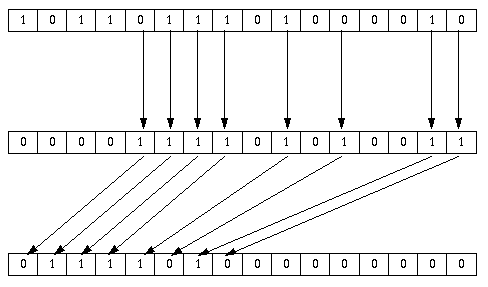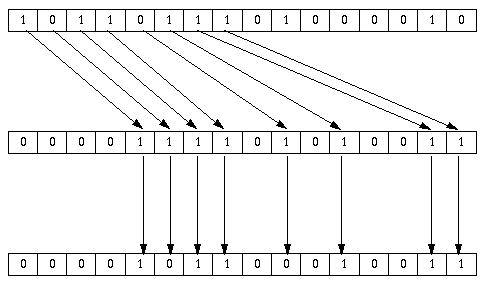# BESM-6 Instruction Set

#### CPU resources visible to the user

(Traditionally, the bits in registers are numbered right to left, starting with 1.)

• 15 15-bit index registers, or modifiers, as they were also called (M to M, the latter might be used as stack register)

• 48-bit accumulator (A):

 48 42
41  40 1
Exponent Sign (pos=0, neg=1) Mantissa (2-complement)
Value = (0.Mantissa - Sign) * 2^(Exponent-64)

• 48-bit "young bits" register (Y): used as extension of A for arithmetic (allowing effective implementation of double precision floating point with 80-bit mantissa), and certain logical instructions

• 6-bit AU mode register (R):

6  5 3
2 1
Suppress FPE ω (omega) mode Suppress rounding Suppress normalisation

ω mode could have the following values:

• 1xx - last instruction was "additive"
• 01x - last instruction was "multiplicative"
• 001 - last instruction was "logical"
• 000 - this mode could only be set explicitly and had no useful meaning

• 15-bit address modification register (C)

### Instruction formats:

All instructions are 24 bit wide and are packed into words in pairs. The branches can be made to word boundaries only.

There are two formats:

Format 1
 24 21
20 19
 18 13
 12 1
M 0 S OPCode1 offset
EA = ([M] + offset + S * 070000 + C) mod 0100000

Format 2
 24 21
20
 19 16
 15 1
M 1 OPCode2 offset
EA = ([M] + offset + C) mod 0100000

### Opcode descriptions

Traditionally, the opcodes in opcode lists are written in octal including bits 20 to 13 or 20 to 16 and assuming S = 0, therefore the opcode ranges are 000 to 077 for format 1 and 20 to 37 for format 2.

The following mnemonics are used in descriptions:

• A - accumulator
• C - address modification register
• E - A[48:42] (exponent)
• M - index register denoted by the M field of the current instruction
• R - AU mode register
• U - EA of the current instruction
• K - program counter
• N - U[7:1]
• V - EA of the current instruction less M (that is, offset + C)
• I - index register denoted by U[4:1] of the current instruction
• J - index register denoted by V[4:1] of the current instruction
• X - memory location determined by the EA of the current instruction
• W - X[15:1]
• Y - "young bits" register
• Z - Y[40:1]

The opcode descriptions are too brief to be exact. The behavior of most arithmetic instructions depends on normalisation and rounding mode, and the behavior of branch instructions depends on the value of the ω mode. For instructions setting additive or multiplicative ω mode, A and X are considered floating point (unless said otherwise), for logical instructions - unsigned integers.

More precise descriptions will follow.

The way register Y is used, although seems bizarre at first, is to simplify the implementation of double precision arithmetic (the second word of a double precision value holds LSBs of mantissa and MSBs of exponent).

OpcodeMnemonicDescriptionFormulaω mode
000ATXStoreX = A;Kept
001STXPopX = A; A = mem[--M]; Logical
002MODModify privileged registers (kernel)Log/Kept
003XTSPushmem[M++] = A; A = X;Logical
006X-AReverse subtract[A,Z] = X - A;Additive
007AMXSubtract absolute values[A,Z] = abs(A) - abs(X);Additive
011AAXBitwise ANDA = A & X; Y = 0;Logical
012AEXBitwise Exclusive ORY = A; A = Y ^ X;Logical
013ARXCyclical add(unsigned) A += (unsigned)X; if (carry) (unsigned)A++; Y = 0;Multipl.
014AVXNegateif (X < 0) A = -A; Y = 0;Additive
015AOXBitwise ORA = A | X; Y = 0;Logical
016A/XDivideA = A / X; Y undefined;Multipl.
017A*XMultiply[A,Z] = A * X;Multipl.
021AUXUnpack bits in A using mask XLogical
022ACXPopulation countA = popcount(A) + X; Y = 0;Logical
023ANXFind highest set bitA = high_1_and_rest(A) + XLogical
024E+XAdd exponentE += X[48:42] - 64; Y = 0;Multipl.
025E-XSubtract exponentE -= X[48:42] - 64; Y = 0;Multipl.
026ASXShiftY = 0; if (X[48:42] >= 64) [A,Y] >>= X[48:42]-64; else [Y,A] <<= 64-X[48:42];Logical
027XTRSet mode registerR = X[47:42];As set
030RTEGet mode registerE = R;Used
031YTAGet young bits registerA = yta (Y, ω mode);Used
032EXTAccess external I/O registers (kernel)Kept
033---
034E+NAdd exponent immediateE += N - 64; Y = 0;Multipl.
035E-NSubtract exponent immediateE -= N - 64; Y = 0;Multipl.
036ASNShift immediateY = 0; if (N >= 64) [A,Y] >>= N-64; else [Y,A] <<= 64-N;Logical
037NTRSet mode register immediateR = N[6:1];As set
040ATISet index registerI = A[15:1];Kept
041STIPop into index registerI = A[15:1], A = mem[--M];Logical
042ITAGet index registerA = I;Logical
043ITSPush index registermem[M++] = A, A = I;Logical
044MTJMove index registerJ = M;Kept
046Unused, but identical to 044 and 045, respectively
047
050
.
.
.
077
*nnExtra-codes, used as system calls and math library functionsVaries or undefined
20
21
22UTCSet C register immediateC = U; ("UTC 0" == NOP)Kept
23WTCSet C registerC = X[15:1];
24VTMSet index register immediateM = V;
25UTMAdd to index register immediateM += V; (or M = U;)
26UZABranch if ω == 0Y = A; if (omega() == 0) K = U;Used
27U1ABranch if ω != 0Y = A; if (omega() != 0) K = U;
30UJUnconditional branchK = U;Kept
32IJReturn from interrupt (kernel)
33STOPStop (unprivileged :-) )
34VZMBranch if index is zeroif (M == 0) K = V;
35V1MBranch is index is not zeroif (M != 0) K = V;
36Unused, but identical to 34
37VLMLoopif (M != 0) { M++; K = V; }Kept

All instructions except UTC and WTC reset C to 0 after execution.

If V of the current instruction is equal to 0 and the index register being used is 017, the following instructions are executed in stack mode:

• ATX,
• XTA,
• A+X,
• A-X,
• X-A,
• AMX,
• AVX,
• AAX,
• AOX,
• AEX,
• APX,
• AUX,
• ANX,
• ACX,
• A*X,
• A/X,
• ARX,
• WTC
that is, M++ is performed after ATX, and M-- is performed before all remaining instructions. To clarify things: the accumulator is the stack top, the stack grows toward bigger addresses, and the stack pointer points to the first available element of the stack.

(It is left to the reader to figure out the semantics of `STX ` and `XTS `.)

#### Finding the number of the highest 1 bit set

```high_1_and_rest(word48 a) {
int bit = 1;
if (a == 0) {
Y = 0;
return 0;
}
while (!(a & 0x800000000000)) {
bit++;
a <<= 1;
}
Y = a << 1; /* the rest of A */
return bit;
}
```

The original instruction set manual assumes a slightly simpler algorithm and says that A = 1 and A = 0 yield the same value 48, "which is somewhat unfortunate"; but in fact all the machines made (even the first one) had the instruction implemented the "correct" way.

#### Pack instruction illustration

A#### Unpack instruction illustration

A#### Semantics of conditional branches

For the UZA and U1A instructions, the ω condition is calculated as follows:

```switch (ω mode) {
case additive: ω = (A != 0); /* A < 0 */ break;
case multiplicative: ω = (A != 1); /* abs(A) < 0.5 */ break;
case logical: ω = (A[48:1] != 0); /* A != 0 */ break;
case 0: ω = 1;
}```

#### YTA semantics

If the current ω mode is "logical", then A = Y; else A[40:1] = Z, A = 0, E += N - 64;